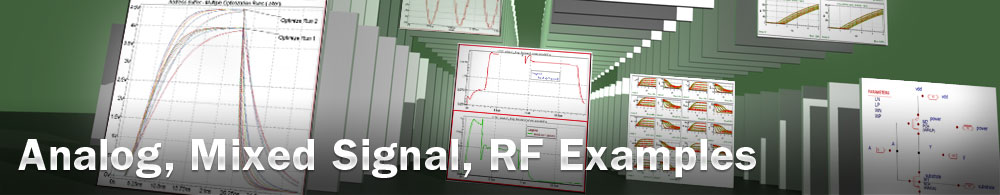Inductor Macro Model Extraction

## opt_ex08 : Inductor Macro Model Extraction

Requires: Utmost IV, SmartSpice, SmartView

Minimum Versions: Utmost IV 1.10.6.R, SmartSpice 4.10.2.R, SmartView 2.28.2.R

This example describes how to extract a simple hybrid Pi macro=model for an RF inductor. The schematic for the hybrid Pi model is shown in . To extract the model, a typical s-parameter measurement is required over the frequency range of interest.

The hybrid Pi inductor macro model is a simple inductor model containing an inductance (Lind) and resistance (Rind) in series which model the low frequency behaviour of the device. The capacitance Cind models the resonant frequency of the inductor which is in parallel to the series resistance and inductance, and the four remaining components model the parasitic losses of the device.

The project file opt_ex08.prj and the data file opt_ex08.uds for this example should be loaded into your database. When opened, the project will look as shown in and when plotted, the example measured data will look as shown in . Included in the measured data are some calculated data representing the ideal inductance and quality factor for the inductor being characterised. The formulae used to calculate these values are as follows;

Ind = y21i / (2 * 3.14159 * freq * y21m*y21m)

Q = (-1) * y11i / y11r

The optimization sequence, which fully automates the extraction of this hybrid Pi inductor macro model example, has three sections.The first section takes the low frequency calculated inductance data and extracts the value of the series inductance. The second section takes the low frequency data and extracts the value of the series resistance of the device. The third section looks at all of the data including the high frequency data and extracts the parasitic loss and resonant circuit element values.

On completion of the sequence, the measured versus simulated characteristics should be as shown in . The model params for the individual hybrid Pi circuit elements can then be exported into an external model library file as shown in the output file opt_ex08.lib. You also have to construct a subcircuit representing the netlist which was used for the model parameter extraction. The netlist for the hybrid Pi model is as shown in .